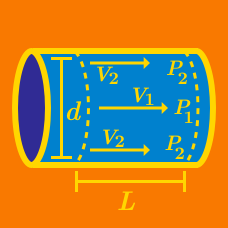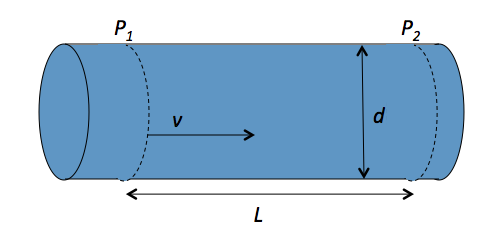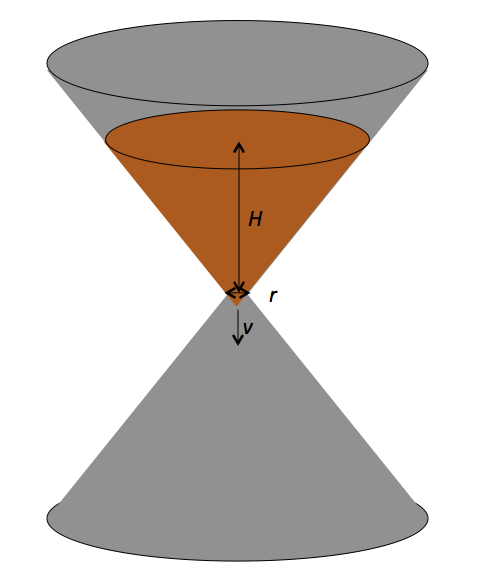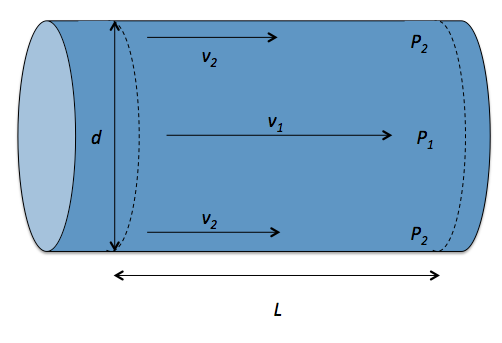Classical Mechanics

# Buckingham Pi Theorem (Dimensional Analysis)

Using the Buckingham $\pi$ theorem, find the formula for the radius of a black hole in term of the black hole’s mass $m,$ the gravitational constant $G,$ and the speed of light $c$As shown in the above figure, a liquid with density $\rho$ and viscosity $\mu$ flows through a pipe with diameter $d.$ In a section of the pipe with length $L,$ the liquid flows with a speed of $v.$ Using the Buckingham $\pi$ theorem, determine the pressure difference $\Delta P = P_1 - P_2$ in terms of the fluid properties $d, L, \rho, \mu$ and $v .$

Details

• the function $f$ is unitless, so that it outputs a pure number.The sand timer in the above figure has a due time of $T.$ The radius of the hole is $r,$ the initial height of the sand is $H,$ and the density of the sand is $\rho.$ Using the Buckingham $\pi$ theorem, determine $T$ in terms of the properties $r, H, \rho,$ and the gravitational constant $G .$As shown in the above figure, a liquid with density $\rho$ flows through a pipe with diameter $d.$ In a section of the pipe with length $L,$ the liquid flows with a speed of $v_1$ at the center and $v_2$ at the edge of the flow. The pressures are $P_1$ at the center and $P_2$ at the edge. Using the Buckingham $\pi$ theorem, express the viscosity $\mu$ of the fluid in terms of the liquid properties $d, L, \rho, \Delta v = v_1 - v_2$ and $\Delta P = P_1- P_2 .$

The drag force $F$ depends on four quantities: two parameters of the cone which are the speed of the cone $v$ and the size of cone $r,$ and two parameters of the air which are the density of the air $\rho$ and the viscosity of the air $\mu.$ Find the independent dimensionless groups that can be produced with $F, v, r, \rho$ and $\mu .$

×